/
/
/
86. The maximum possible value for the coefficient of static
Not my Question
Flag Content

# Question :   86. The maximum possible value for the coefficient of static : 2102097

86. The maximum possible value for the coefficient of static friction is:

A. 0.50.

B. 1.00.

C. a value up to but not quite 1.00.

D. greater than 1.00.

87. A box is to be moved across a level surface. A force of magnitude 200 N may be applied at an angle of 30? below the horizontal to push the box or at an angle of 30? above the horizontal to pull the box, either application sufficient to overcome friction and move the box. Which application will cause the box to have the greater acceleration?

A. the one below the horizontal

B. the one above the horizontal

C. both give equal acceleration

88. A crate is in the bed of a pickup truck on a level road. The coefficients of static friction and kinetic friction between the crate and the bed of the truck are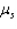and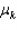, respectively. When the truck starts from rest, what is the limiting acceleration that it can have before the crate starts sliding along the truck bed?

A.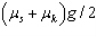B.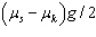C.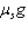D.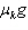89. Two boxes are stacked on top of one another on the back of a flat bed truck on a level roadway. The coefficient of static friction between the boxes is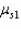, and the coefficient of static friction between the bottom box and the bed of the truck is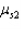. What is the limiting acceleration the truck can undergo before one or both of the boxes start sliding if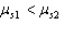?

A.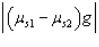B.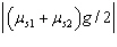C.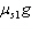D.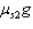90. Two blocks are at rest on a horizontal frictionless surface with a compressed spring between them. The 10-kg block accelerates when the string holding the system in compression is cut and the 20-kg box accelerates in the opposite direction. What is the magnitude of the force from the expanding spring on the 20-kg box when the 10-kg box is undergoing an acceleration of 8.0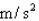?

A. 40 N

B. 160 N

C. (160/3) N

D. 80 N

## Solution 5 (1 Ratings )

Solved
Physics 1 Year Ago 91 Views